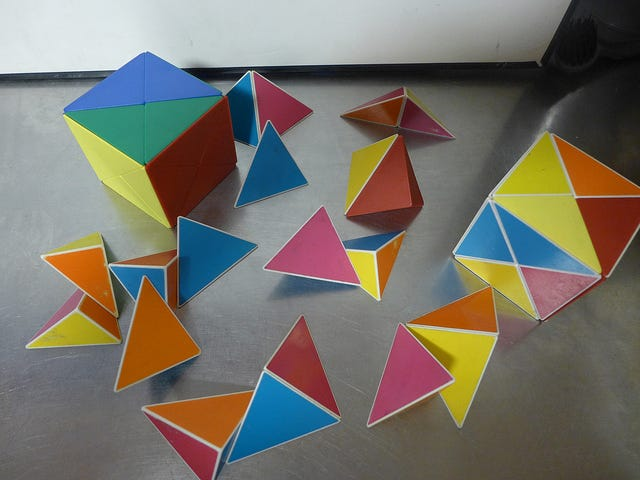playstation

# A Philosophical Investigation

## In the Wittgensteinian Tradition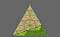6 x 2 = 12 (geen); 6 x 4 = 24 (tan). 12 + 24 = 36 = 6 x 6 (whole)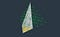2 x 2 x 5 = 20; 2/5 x 2/5 x 1 = 4/25.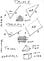Anthropologist’s Notebook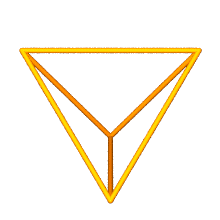Cube of Volume 3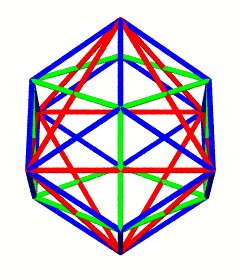Cube + Octahedron (dual) = Rhombic Dodecahedron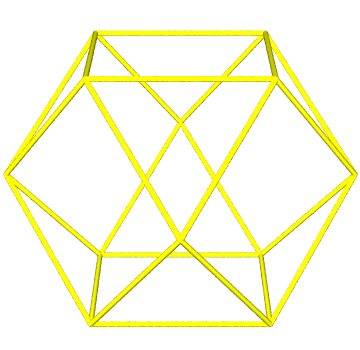jitterbug transformation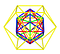Nested Polys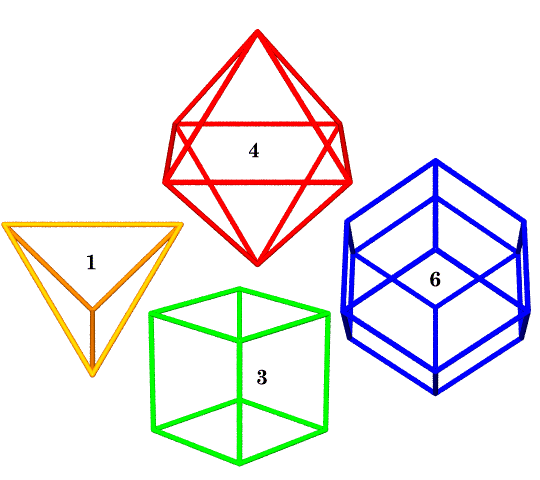canonical volumes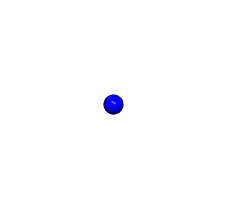1, 12, 42, 92… 10 F F + 2 (where F = intervals between spheres along an edge)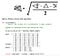from the School of Tomorrow curriculum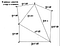S Module as Plane Net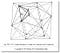S module in situ